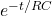## Since you analyzed the charging of a capacitor for a DC charging voltage, how is it possible that you

Question

Since you analyzed the charging of a capacitor for a DC charging voltage, how is it possible that you

in progress 0
6 months 2021-08-13T16:54:32+00:00 1 Answers 10 views 0

## Answers ( )

I = E/R   e^{-t/RC}

Explanation:

In a capacitor charging circuit you must have a DC power source, the capacitor, a resistor, and a switch. When closing the circuit,

E -q / c-IR = 0

we replace the current by its expression and divide by the resistance

I = dq / dt

dq / dt = E / R  -q / RC

dq / dt = (CE -q) / RC

we solve the equation

dq / (Ce-q) = -dt / RC

we integrate and evaluate for the charge between 0 and q and for the time 0 and t

ln (q-CE / -CE) = -1 /RC   (t -0)

eliminate the logarithm

q – CE = CEq = CE (1 + 1/RC  e^{-t/RC} )

In general the teams measure the current therefore we take the derivative to find the current

i = CE (e^{-t/RC} / RC)

I = E/R   e^{-t/RC}

This expression is the one that describes the charge of a condensate in a DC circuit